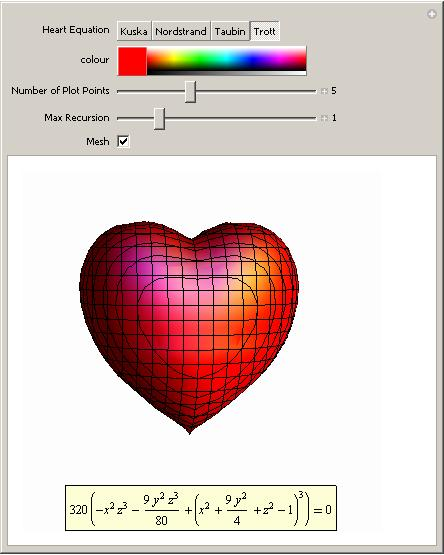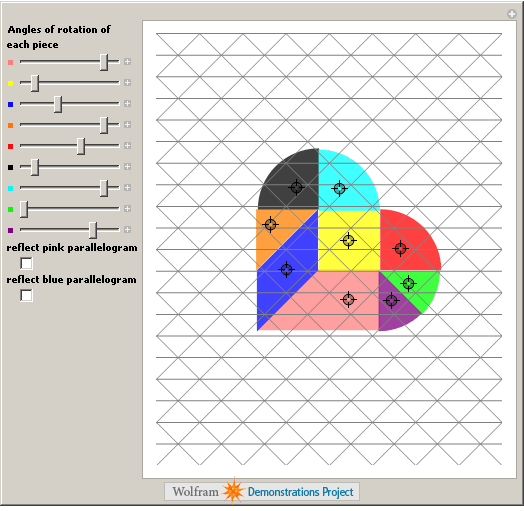## A little mathematics for Valentine’s day

February 10th, 2010 | Categories: general math | Tags:

This is not a serious post but then mathematics doesn’t have to be serious all the time does it?  Ever wondered what the equation of a 3D heart looks like?  An old post of mine will help you find the answer using Mathematica.  Click on the image for more details (and a demonstration that you can run using the free Mathematica player).I don’t save all of my love for Mathematica though. I’ve got some for MATLAB too:

%code to plot a heart shape in MATLAB
%set up mesh
n=100;
x=linspace(-3,3,n);
y=linspace(-3,3,n);
z=linspace(-3,3,n);
[X,Y,Z]=ndgrid(x,y,z);
%Compute function at every point in mesh
F=320 * ((-X.^2 .* Z.^3 -9.*Y.^2.*Z.^3/80) + (X.^2 + 9.* Y.^2/4 + Z.^2-1).^3);
%generate plot
isosurface(F,0)
view([-67.5 2]);


Did you know that the equation for a heart (or a cardioid if you want to get technical) is very similar to the equation for a flower?  The polar equation you need isand you get a rotated cardioid for n=1.  Change n to 6 and you get a flower.  Let’s use the free maths package, SAGE, this time.

First, define the function:

def eqn(x,n):
return(1-sin(n*x))


then plot it for n=1

polar_plot(eqn(x,1), (x,-pi, pi),aspect_ratio=1,axes=False)and for n=7

polar_plot(eqn(x,7), (x,-pi, pi),aspect_ratio=1,axes=False)Back to Mathematica and the Wolfram Demonstrations project. We have a Valentine’s version of the traditional Tangram puzzle.Feel free to let me know of any other Valentine’s math that you discover, puzzles, fractals or equations, it’s all good :)

Update Feb 14th 2011

Mariano Beguerisse Díaz sent me some MATLAB code that uses a variation on the standard mandelbrot and I turned it into the movie below.  His original code is in the comments section

1. I have a ‘fuzzy’ Mandelbrot fractal in MATLAB
Just run this script, have a go!

% Fuzzy heart-shaped Mandelbrot fractal
% Mariano Beguerisse
% October 2010

% iterations
n = 300;
% resolution
N = 200;
% Create grid
x = linspace(-1, 1, N);
y = linspace(-1.4, .6, N);
[X,Y] = meshgrid(x, y);

Z = X + i*Y;
Zn = Z;

figure
for j=1:n
% Mandelbrot map with random noise
Zn = -i*(Zn).^2 +  (rand(N,N).^(1/5)).*Z;
M = abs(Zn);
ind1 = find(M<2);
ind2 = find(M>=2);
M(ind1) = 0;
M(ind2) = -1;
m = 1;
imagesc(x, y, (M/m))
colormap([1 1 1; 1 0 0])
set(gca,'YDir','normal')
title(n-j)
pause(0.01)
end
title('Love or whatever', 'fontsize', 16)


2. That’s nice :)